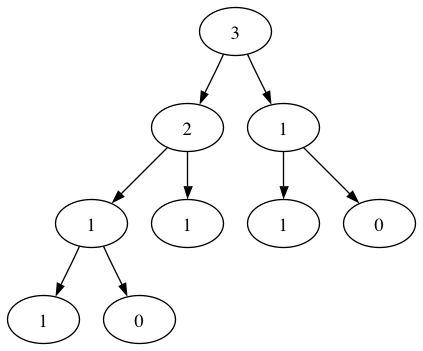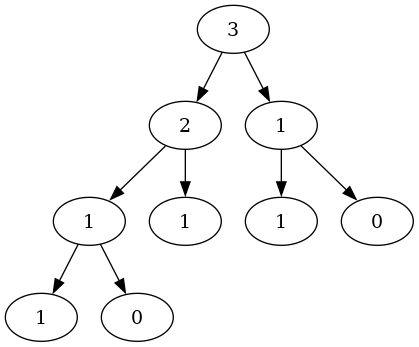# Is it possible to graph the Fibonacci sequence?

Hello,

I would like to replicate this simple graph:I’ve been banging my head trying to find something that works. The problem comes from duplicated labels which I’d like to use. I’ve played with the ordering param, but it doesn’t help for this use case unfortunately.

``````strict digraph G {
graph [ordering = "out";];
// level 1
3 -> 2;
3 -> 1;

// level 2
2 -> 1;
2 -> 1;
1 -> 1;
1 -> 0;

// level 3
1 -> 1;
1 -> 0;
}
``````

Is it possible to do this simply with Graphiz? I’ve managed to get something that sort of works, like this:

``````digraph {
// level 0
fib4 [label = "fib(4)";];
// level 1
fib3__1 [label = "fib(3)";];
fib2__1 [label = "fib(2)";];
// level 2
fib2__2 [label = "fib(2)";];
fib1__2 [label = "fib(1)";];
fib1__2a [label = "fib(1)";];
fib0__2b [label = "fib(0)";];

// level 1
fib4 -> fib3__1;
fib4 -> fib2__1;

// level 2
fib3__1 -> fib2__2;
fib3__1 -> fib1__2;
fib2__1 -> fib1__2a;
fib2__1 -> fib0__2b;
}
``````

But doing this becomes quickly unmanageable.

Thanks!

I understand the “starting” problem that node labels have to be unique. I am not sure if that is the only problem you are encountering, so let’s go forward.

• Do you want a naming scheme that has “meaning”, and if so how much meaning?
• We could use random words to name the nodes (e.g. betty, bob, ball, broom, …) [cute, but a pain to implement if the graph gets to be of any size]
• Or, a scheme that identifies row (rank) and position within that row (e.g. a1, b1, b2, c1, c2, c3, …) [this seems pretty easy to use, but the “meaning” is not obvious]
• Or a scheme that is based on “heritage” with each name showing its parents (e.g. 3, 3_2, 3_1, 3_2_1a, 3_2_1b, …) [OK, maybe not exactly a great scheme, but something to indicate the parentage]
• All-in-all I like the second scheme
• Are there other problems, maybe with layout?

Here is scheme #2, created manually.
Note that this syntax `a1 -> {b1 b2}` is not mandatory, but it helps me visualize the binary tree structure as I wrote the input:

``````digraph fib {
a1 -> {b1 b2}
b1 -> {c1 c2}
b2 -> {c3 c4}
c1 -> {d1 d2}

a1 [label=3]

b1 [label=2]
b2 [label=1]

c1 [label=1]
c2 [label=1]
c3 [label=1]
c4 [label=0]

d1 [label=1]
d2 [label=0]
}
``````

Giving:Not to state the obvious, but isn’t this inevitable? Fibonacci is a recursive algorithm that results in numerous duplicate calls. I would assume if you want to graph something like this, you would programmatically generate the graph.

Thanks @steveroush! I like your method it’s very easy to follow.

I’ve been having trouble with a recursive algorithm so I thought I’d try to use graphiz to sketch out my thinking.

I took the Fibonacci sequence as an example, because if I reckoned if I could sketch it out easily, then I would be able to construct more complicated graphs.

That’s basically what I wanted to draw (though I’m not sure my thinking is 100% correct here)Pretty cool toolAh I see. To complete my thought and in case it helps your thinking, here is something that I believe implements fibonacci while also printing the Graphviz spec you want:

``````node_counter = 0

def fibonacci(n: int, my_id: int = 0) -> int:

global node_counter

print(f'  n{my_id}[label="{n}"];')

if n == 0:
return 0
if n == 1:
return 1

child1_id = node_counter + 1
node_counter += 1
print(f"  n{my_id} -> n{child1_id};")
child1 = fibonacci(n - 1, child1_id)

child2_id = node_counter + 1
node_counter += 1
print(f"  n{my_id} -> n{child2_id};")
child2 = fibonacci(n - 2, child2_id)

return child1 + child2

print("digraph {")
fibonacci(4)
print("}")
``````

Very nice!

You said it but I missed it: it didn’t occur to me that I could generate a graph pretty simply like that.

I’ll use that technique as a complement to analyze my code.

Thanks!

Having wistful feelings about alternative tree layout algorithms.

I thought there was a “todo” entry for trees, but can’t find any open issue for a tree layout engine / pre-processor / language add-on.
It strikes me (naively) that the hardest part is the requirements.
Anybody want to have a go at creating an issue?